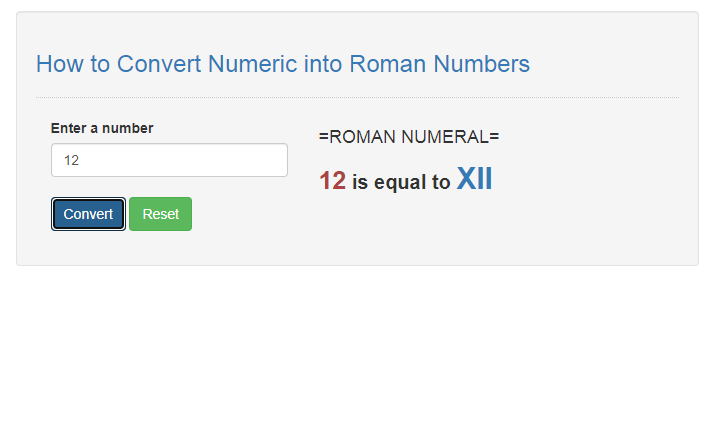# How to Convert Numeric into Roman Numbers in JavaScript

## Introduction

In this tutorial we will create a How to Convert Numeric into Roman Numbers in JavaScript. This tutorial purpose is to teach you how to you can convert numeric into roman numbers. This will cover all the basic function will change your background I will provide a sample program to show the actual coding of this tutorial.

This tutorial is simple and easy to understand just follow the instruction I provided and you can do it without a problem. This program can be use to any application that uses numbers for displaying result. I will give my best to provide you the easiest way of creating this program Convert numeric to roman numeral. So let's do the coding.

## Before we get started:

This is the link for the template that i used for the layout design https://getbootstrap.com/.

## Creating The Interface

This is where we will create a simple interface to our application. This code will only display the textbox and buttons in the form. To create this simply copy and write it into your text editor, then save it as index.html.
`<!DOCTYPE html><html lang="en">	<head>			<meta charset="UTF-8" name="viewport" content="width=device-width, initial-scale=1" />		<link rel="stylesheet" type="text/css" href="css/bootstrap.css"/>		<script type="text/javascript" language="Javascript" src="code_js.js"></script>	</head><body>	<nav class="navbar navbar-default">		<div class="container-fluid">			<a class="navbar-brand" href="https://sourcecodester.com">Sourecodester</a>		</div>	</nav>	<div class="col-md-3"></div>	<div class="col-md-6 well">		<h3 class="text-primary">How to Convert Numeric into Roman Numbers</h3>		<hr style="border-top:1px dotted #ccc;"/>		<div class="col-md-5">			<div class="form-group">				<label>Enter a number</label>				<input type="number" id="number" class="form-control"/>				<br />				<button class="btn btn-primary" onclick="convertToRoman();">Convert</button>				<button class="btn btn-success" onclick="reset();">Reset</button>					</div>		</div>		<div class="col-md-6">			<h4>=ROMAN NUMERAL=</h4>			<div id="result"></div>		</div>	</div><script src="script.js"></script></body></html>`

## Creating JavaScript Function

This is where the main function of the application is. This code will convert the entered numeric into roman number when the button is clicked. To do this just copy and write these block of codes inside the text editor and save it as script.js.
`function numericToRoman(val) {	var roman=['I', 'IV', 'V', 'IX', 'X', 'XL', 'L', 'XC', 'C', 'CD', 'D', 'CM', 'M'];	var numbers=[1, 4, 5, 9, 10, 40, 50, 90, 100, 400, 500, 900, 1000];	var x = parseInt(val); 	if( x<1 || x>4999 ){		alert(x+' is invalid number.');		return 0;	}	var result = "";	for(i=12; i>=0;)		if(numbers[i]<=x ){			result += roman[i];			x -= numbers[i];		}	 else{		i--;	}	return(result);} function convertToRoman(){	if(document.getElementById('number').value != ""){		var number = parseInt(document.getElementById('number').value); 		if(numericToRoman(number) !== 0){			document.getElementById('result').innerHTML = "<label style='font-size:20px;'><span style='font-size:25px;' class='text-danger'>"+number+"</span> is equal to <span style='font-size:30px;' class='text-primary'>"+numericToRoman(number)+"</span></label>";		}else{			document.getElementById('result').innerHTML = "";		}	}else{		alert("Please enter a number first!");	} } function reset(){	document.getElementById('number').value = "";	document.getElementById('result').innerHTML = "";}`

In the code above we first create a method called numericToRoman(), this function will set all the equal values of the roman numbers to numeric values. This method uses several functionalities including loops and conditional statement in order to match the number you have entered. And next we will create a method that will display the pre-convert numeric number into the html page content, we will call this method convertToRoman().

That' its we just successfully create the application that can convert the numeric into roman numbers, try to run the application and see if it works.

## Output: